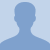# Number of SolutionsEquations can have different numbers of solutions and some come from real numbers while others come from imaginary numbers. In this video lesson, Mr. Howell will take you through the different number of solutions of equations... Equations can have different numbers of solutions and some come from real numbers while others come from imaginary numbers. In this video lesson, Mr. Howell will take you through the different number of solutions of equations and how to find them.
More... Collapse
107 Views